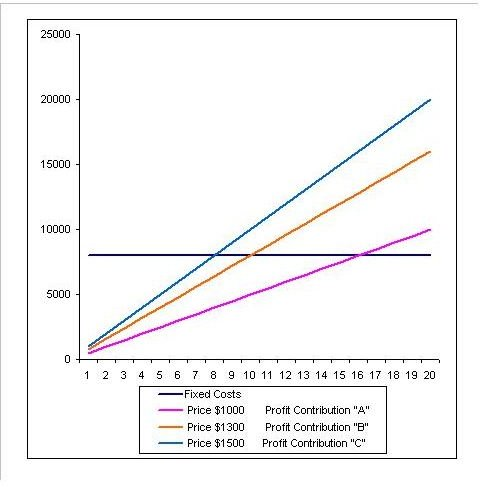# How to Use a Break-Even Analysis Excel Template

Page content

When companies want to determine the ideal quantity that must be sold to cover expenditures, they perform a break-even analysis, often with the help of an Excel template. A break-even analysis is a simple approach that uses a company’s fixed and variable costs, as well as its product’s or service’s price, in order to determine the quantity that must be sold to cover these aforementioned costs.

## What Is Involved in a Break-Even Analysis?

When companies perform a break-even analysis, they must know their existing fixed and variable cost structure, as well as the selling price of their products or services. Fixed costs are a company’s salaries, its rent and any fixed cost that a company pays monthly or yearly. Variable costs pertain to the usage of parts and materials needed to manufacture a given volume of product. They include the product’s material and labor. To make this example as complete as possible, we’ll assume the following variables for a fictitious company’s fixed and variable costs, as well as the price of their product.

• Total Fixed Costs = \$8000.00
• Total Variable Costs = \$500.00 per unit
• Product or Service Price = \$1000.00 per unit

The break even quantity calculation is expressed as follows:

• Break Even Quantity = BEQ

• Fixed Costs = F = \$8000.00

• Variable Costs = V =\$500.00

• Price = P = \$1000.00

• BEQ = F/(P-V)

• BEQ = \$8000.00 / (\$1000.00 - \$500.00)

• BEQ = \$8000.00 / \$500.00

• Break even quantity is 16

## Understanding the Profit Contribution of the Break Even Analysis

When companies use a break-even analysis Excel template, they must pay close attention to the profit contribution of each and every product or service sold. In our example above, the profit contribution is \$500.00 and is calculated by taking the price of the product (\$1000.00) minus the variable costs of the product (\$500.00). This profit contribution is a pivotal aspect within the break even analysis.

In the following break-even analysis, there are two additional prices the company is considering charging for its product. There’s the original price of \$1000.00 as outlined in our example above, and two additional prices of \$1300.00 and \$1500.00. While we’ve figured out the break even point based on the \$1000.00 sell price to be 16 units, we’ll now calculate the break even point for the other two prices.

Break even quantity with a sell price of \$1300.00

• BEQ = F/(P-V)
• BEQ = \$8000.00 / (\$1300.00 - \$500.00)
• BEQ = \$8000.00 / \$800.00
• Break even quantity is 10 units

Break even quantity with a sell price of \$1500.00

• BEQ = F/(P-V)
• BEQ = \$8000.00 / (\$1500.00 - \$500.00)
• BEQ = \$8000.00 / \$1000.00
• Break even quantity is 8 units

These different prices are represented in the graph below. The “blue” line represents the break even quantity where the profit contribution is \$1000.00 and where the product’s price is \$1500.00. The “orange” line represents the break even quantity where the profit contribution is \$800.00 and corresponds to the \$1300.00 price. Finally, the “purple” line represents the profit contribution of \$500.00 from our original price of \$1000.00.When looking at the graph, it’s important to note that the break even points occur when the combined profit contribution, from each unit sold, meets the company’s fixed costs. Anything below these intersection points is considered a loss for the company. Consequently, anything above these intersection points is considered profit.

It’s never as simple as just raising the price of a product. In this break even analysis excel template, we’ve shown how different prices will allow a fictitious company to achieve break even sooner. A given price must match the market’s and customer’s threshold for pricing tolerance. However, companies can achieve similar results by reducing both their fixed and variable cost structures. Reduce these costs, and the company is able to reach break even sooner.

Image Credit: https://www.morguefile.com/archive/display/702627